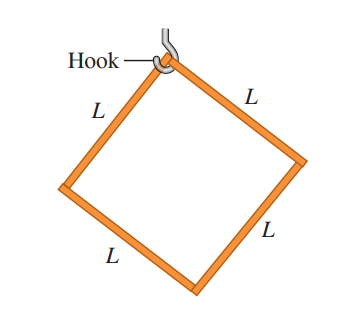### Square SHM

##### Score: 3 Points

A square object of mass $m$ is constructed of four identical uniform thin sticks, each of length $L$, attached together. This object is hung on a hook at its upper corner. If it is rotated slightly to the left and then released, at what time period will it swing back and forth? Consider $L= 1m$, $m=2kg$ and $g=9.8 m/s^2$Simple Harmonic Motion

#### Statistics

Tried 54

Solved 6

First Solve @Pial-age_what